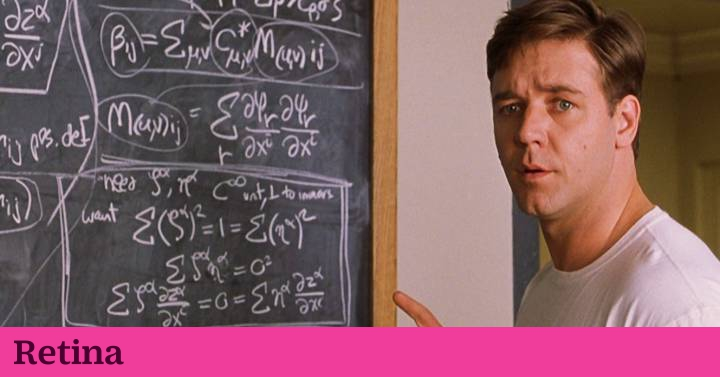June 21, 2021

# Facebook trains an artificial intelligence capable of solving equations | InnovationAlgorithms are good at adding, subtracting and multiplying whole numbers, but doing mathematical operations such as differential or integral equations is still a challenge for them. At least, for most of them. Facebook researchers AI Research in Paris (France) have managed to develop an algorithm capable of doing so in just a few seconds. For the first time, they have trained a neural network to be able to do the symbolic reasoning necessary to differentiate and integrate mathematical expressions. “His work represents an important step towards a more powerful mathematical reasoning and a new way to apply neural networks beyond traditional pattern recognition tasks, “says the publication MIT Technology Review.

Neural networks are capable of very efficiently performing diverse tasks based on specific skills such as pattern, face and object recognition, data analysis, certain types of natural language processing and even games like chess. But until now, no one had been able to train them to solve complex mathematical problems.

One of the greatest difficulties with mathematics, both for humans and for machines, is the abbreviations that are used. “For example, the expression x3 It is an abbreviated way of writing x multiplied by x multiplied by x. In this example, multiplication is the abbreviation of the repeated sum, which is itself the abbreviation of the total value of two quantities together, “they explain in MIT Technology Review. If neural networks do not understand these abbreviations, there is little chance that they will learn to use it. The same goes for people.

So researchers Guillaume Lample and François Charton have devised a simple way to break down mathematical abbreviations to make them more understandable. They have done so by representing the expressions as tree-like structures. In this way, the data can be processed by a network called Seq2seq. “Interestingly, this approach is often also used for machine translation, where a sequence of words in one language has to be translated into a sequence of words in another language.” In fact, Lample and Charton stress that their approach basically treats mathematics as a natural language.

The next phase consisted of the training process. The researchers compiled a large database: 80 million examples of first and second degree differential equations and 20 million examples of integrated expressions. In processing this data set, the neural network learned to calculate the derivation or integral of a given mathematical expression. In the end, Lample and Charton tested their neural network with 5,000 expressions that they had never seen before and compared the results created in 500 cases with those of commercially available programs, such as Maple, Matlab and Mathematica.

These programs use a 100 page algorithm for integration only. In many cases, conventional programs cannot find any solution, after trying for 30 seconds. In comparison, the neural network takes about a second to find its solutions. Charton details: “In all the tasks, we observe that our model significantly exceeds Mathematica. In the integration of functions, our model obtains an accuracy close to 100%, while Mathematica barely reaches 85%.”

“As far as we know, no study has investigated the ability of neural networks to detect patterns in mathematical expressions,” Charton explains. The result has enormous potential, according to MIT, although researchers have not revealed Facebook’s plans for this approach. “But it is not difficult to see how it could offer its own symbolic algebra service that outperforms current market leaders.”